MATHEMATICS

 Time: Two Hours (8.30 AM – 10.30 AM) Max.Marks: 60

NOTE:-

1. Attempt all questions.
2. Rough work must be enclosed with answer book.
3. While answering, refer to a question by its serial number as well as section heading. (eg.Q2/Sec.A)
4. There is no negative marking.
5. Answer each of Sections A, B, C at one place.
6. Elegant solutions will be rewarded.
7. Use of calculators, slide rule, graph paper and logarithmic, trigonometric and statistical tables is not permitted.

Note:- All answers to questions in Section-A, Section-B, Section-C must be supported by mathematical arguments. In each of these sections order of the questions must be maintained.

SECTION-A

This section has Five Questions. Each question is provided with five alternative answers. Only one of them is the correct answer. Indicate the correct answer by A, B, C, D, E.

(6x2=12 MARKS)

1. A triangle has integral sides. Its perimeter is 2006. One of the sides is sixteen times another side. The number of such triangles is [ ]

A) 0 B) 1 C) 2 D) 3 E) 4

2. Given that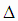ABC is an arbitrary triangle. The number of points P in the interior of the triangle such that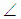APB =BPC =CPA is [ ]

A) 0 B) 1 C) 2

D) not necessarily 0, not necessarily 1, but either 0 or 1

E) not necessarily 1, not necessarily 2, but either 1 or 2

3. The number of rhombi that can be inscribed in a parallelogram such that each side of the parallelogram contains precisely one vertex of the rhombus is [ ]

A) 0 B) 1 C) 0 or 1 D) 0 or 2

E) infinitely many

4. In this question a polynomial means a polynomial with real coefficients. P(x) is a polynomial of degree 2006 and P(x)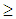0 for all real x. Then [ ]

A) P(x) must have at least one complex root

B) P(x) cannot have 2006 distinct real roots

C) P(x) is a product of 1003 quadratics, none of which has linear factors.

D) P(x) cannot have a polynomial factor of the form (x-k)2005

E) none of these

5. If 0<a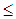xb, 0<ayb and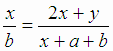, then [ ]

A) a=x=y=b B) a=x<y=b C) a=y<x=b D) a=yx=b

E) none of these

6. p, q, r are given real numbers. Consider the polynomials (q-r)(x-q)(x-r) + (r-p)(x-r)(x-p) + +(p-q)(x-p)(x-q) + k for all real k. Then [ ]

A) there exists a k such that the degree of the polynomial is 2

B) there exists a k such that the degree of the polynomial is 1

C) there exists no k such that the degree of the polynomial is 0

D) there exists no k such that the degree of the polynomial is undefined

E) none of these

SECTION-B

This section has Five Questions. In each question a blank is left. Fill in the blank.

(6x2=12 MARKS)

1. A lattice point is a point both of whose coordinates are integers. A is (0, 0), B is (4012, 6018). If n is the number of lattice points lying on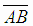, then n = _________

2. Two circles of equal radius r are inscribed in a semicircular sector of radius ‘1’ such that they touch each other externally. Then r = ___________

3. In a triangle ABC, D, E are points on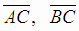respectively such that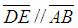and DE = 5. If AB = 8 and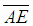bisects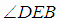, then the length of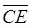= ____________

4. 1 +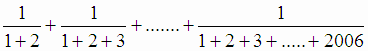= ____________

5. The characteristic of log3 2006 is __________

6. If the positive integer m is such that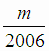is closest to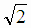, then m = ________

SECTION-C

(6x2=12 MARKS)

1. A(0, 0), B(4012, 0), C(4012, 6018), D(0, 6018) determine a rectangle which is the union of 4012 x 6018 unit squares. Determine the number of these unit squares which have a nonempty intersection with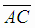.

2. P is a point not lying on a line l. Explain how you construct an equilateral triangle PQR such that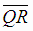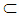l.

3. Let E be the midpoint of the side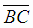ofABC and let F be chosen inso that AC=3FC. Determine the ratio of the areas,ABEF :EFC.

4. Does the equation 2006 = (x+y)2 + 3x + y have a solution in nonnegative integers?

5. Without removing brackets at any stage factorize 2y(y+z)-(x+y)(x+z)

6. Factorize the polynomial in x: (t2+1)x3 – (t2-1)x2 – (t2+1)x + (t2-1)

Section-D

(6x4=24 MARKS)

1. Treating the equalities log102 = 0.3010 and log103 = 0.4771 as exact, prove that the sum to 30 terms of the series 2 + 6+ 18 + 54 + ….. exceeds 2x1014.

2. Factorize a5(b-c) + b5(c-a) + c5(a-b)

3. Prove that (n+1) is either itself a prime or a product of primes for every positive integer n.

4. A triangle ABC is turned about the vertex A in the plane of the triangle into the position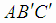. If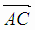bisects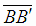, prove that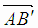bisects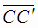.

5. P is a point in the plane of parallel lines l, m. Explain how you locate a point Q on l and a point R on m such that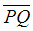and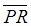are equal in length and perpendicular to each other. Discuss only the case when P lies between l and m.

6. A, B, C are respectively (1002, 1005), (2004, 2001) and (1003, 1000) and P0 is (1, 4). Points Pi, i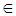N are such that

A is the midpoint of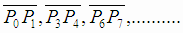B is the midpoint of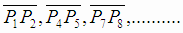C is the midpoint of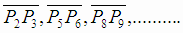Estimate the ratio of lengths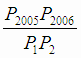.